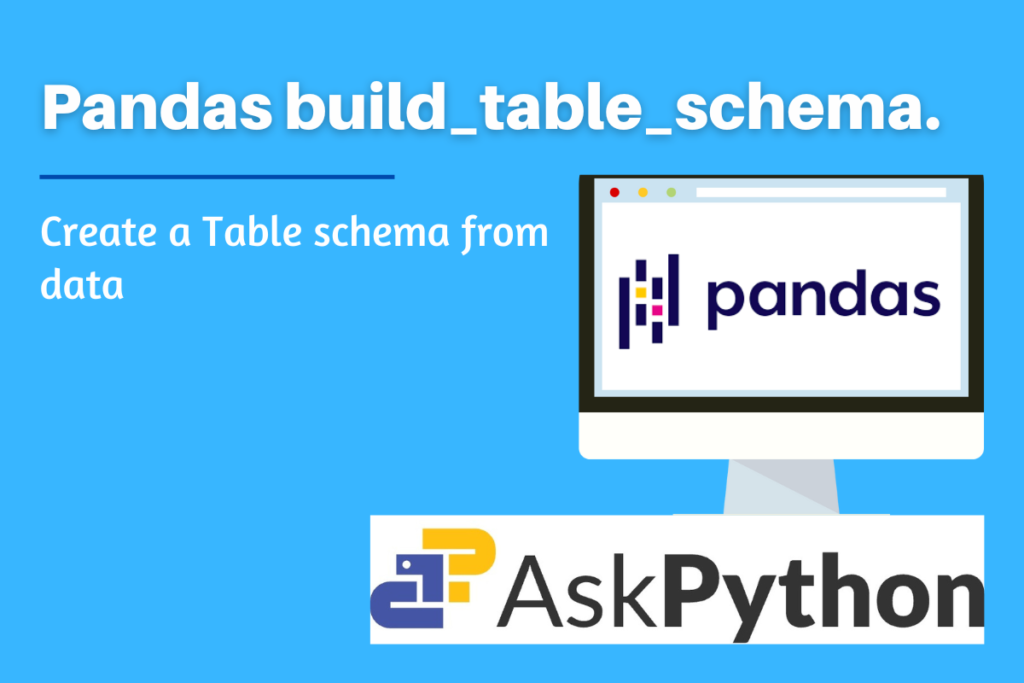# Pandas build_table_schema – Create a Table schema from data.In this article, let’s try to understand `'build_table_schema()'` yet another helpful Pandas Package method. The Pandas software package for the Python programming language is used in the modification of the data as well as in analyzing data.

Both “Panel Data” and “Python Data Analysis” are referred to as “Pandas.” It provides a vast number of methods and data structures for dealing with mathematical tables and time series. It is software that is open-sourced and hence freely available.

The goal of this function is to help in the creation of a table schema for the provided input data. Let us try to understand the use cases, syntax, and implementation of this function in Python Programming Language.

## Why is build_table_schema() used?

This function creates a table schema for given input data. A specification called Table Schema is used to describe tabular datasets as JSON objects. The JSON contains details on the field names, kinds, and additional properties. (To read more about table schema click here.) The output data type for this function is always a dictionary (dict).

### Syntax of build_table_schema()

```pandas.io.json.build_table_schema(data, index=True, primary_key=None, version=True)
```

Parameters:

• data: Series, DataFrame, Required – input data
• index: bool, the default is set as True, Optional – Whether or not to add data.index to the output schema.
• primary_key: bool or None, the default is set as True, Optional – names of the columns that will serve as the primary key. If the index is unique, the default None will set “primaryKey” to the level or levels of the index.
• version: bool, the default is set as True, Optional – Whether or not to include a field pandas version with the Pandas version that most recently changed the table schema. This version might not be the same as the installed Pandas version.

## Implementation of build_table_schema() in Python

Before beginning the methods, be sure to install and load the Pandas package into your IDE.

```import pandas as pd
```

The following dataframe is used for examples:

```df = pd.DataFrame(
{'Col1': ['a','b','c','d'],
'Col2': [1,2,3,4],
'Col3': [1.1, 1.2, 1.3, 1.4]},
index=pd.Index(range(4), name='idx'))

print(df)
```

OUTPUT

### Example 1: Passing only the dataframe as a parameter

```#creating dataframe
df = pd.DataFrame(
{'Col1': ['a','b','c','d'],
'Col2': [1,2,3,4],
'Col3': [1.1, 1.2, 1.3, 1.4]},
index=pd.Index(range(4), name='idx'))

pd.io.json.build_table_schema(df)
```

OUTPUTExample 1: Passing only the dataframe as a parameter (Build Table Schema)

It should be noted that the result/output displays the other parameters because they are all set to True by default. The primary key, meanwhile, is set to the index by default. Also, the first row of the output is an additional row named ‘idx’ and indicates the index for the dataframe. The version parameter is by default set to True, and hence depicted the Pandas package version the dataframe is working on.

### Example 2: Passing other parameters

```df = pd.DataFrame(
{'Col1': ['a','b','c','d'],
'Col2': [1,2,3,4],
'Col3': [1.1, 1.2, 1.3, 1.4]},
index=pd.Index(range(4), name='idx'))

pd.io.json.build_table_schema(df, index = False, primary_key = False, version = False)
```

OUTPUT